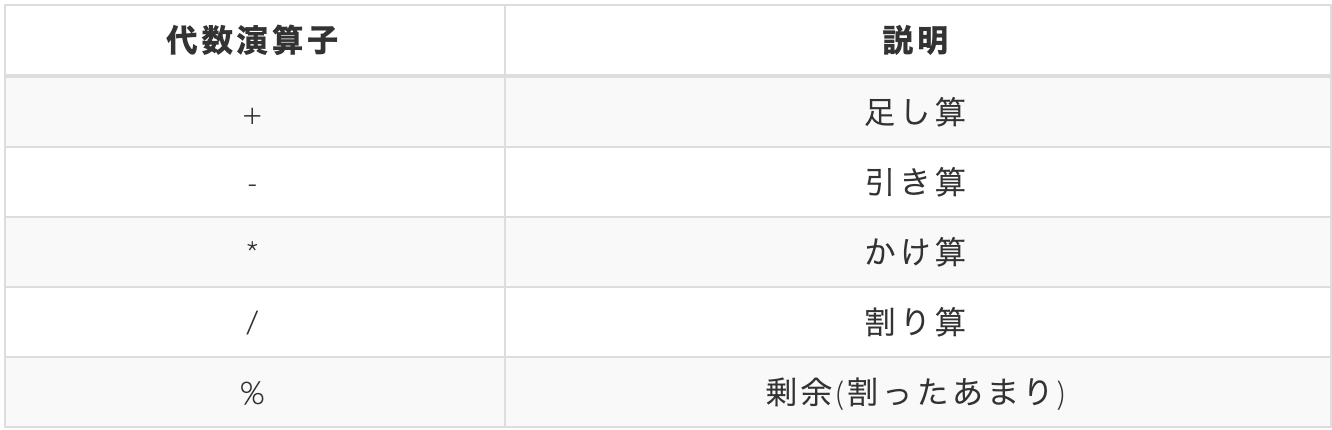# operator

** A symbol used to calculate and compare values in programming. Typical operators in calculation are + </ font> and -</ font>, and typical operators in comparison are <font color = "Red" There are ">> </ font> and << / font>. ** **

By using this simple symbol operator, calculations and comparisons can be performed instantly.

# Algebraic operator

Operators that perform calculations are called algebraic operators. The following operators can be used for general calculations in Ruby.The usage is the same as the calculation of ordinary arithmetic. However, please note that ** the calculation of the remainder is unfamiliar **. For example, you can find the "remainder of 17 divided by 5".

See the example below.

#### `[Example] irb`

``````
irb(main):001:0> 1000 + 2000
=> 3000

#subtraction
irb(main):002:0> 3000 - 1500
=> 1500

#multiplication
irb(main):003:0> 50 * 40
=> 2000

#division
irb(main):004:0> 600 / 15
=> 40

#Residual operation (remainder of 5 ÷ 2)
irb(main):005:0> 5 % 2
=> 1
``````

# Let's run the following code in irb

Let's calculate a few hours a year.

#### `irb`

``````
irb(main):001:0> 24 * 365
``````

When you execute it, the calculation result will be displayed instantly. Please try it! !!

# Summary

** ・ Operators are used for calculation and comparison of values in programming. The symbol used. -Algebraic operators belong to operators and perform calculations among them. ** **